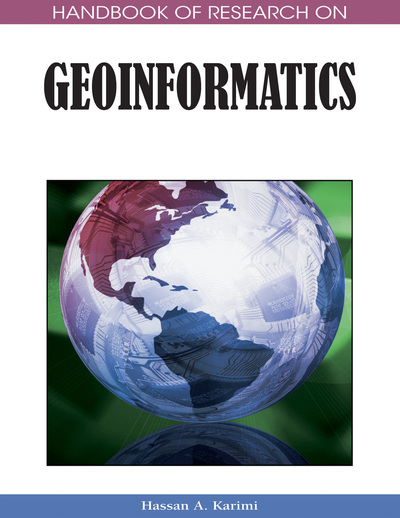# Spatial Interpolation

Xiaojun Yang (Florida State University, USA)
DOI: 10.4018/978-1-59140-995-3.ch017

## Abstract

Spatial interpolation is a core component of data processing and analysis in geoinformatics. The purpose of this chapter is to discuss the concept and techniques of spatial interpolation. It begins with an overview of the concept and brief history of spatial interpolation. Then, the chapter reviews some commonly used interpolations that are specifically designed for working with point data, including inverse distance weighting, kriging, triangulation, Thiessen polygons, radial basis functions, minimum curvature, and trend surface. This is followed by a discussion on some criteria that are proposed to help select an appropriate interpolator; these criteria include global accuracy, local accuracy, visual pleasantness and faithfulness, sensitivity, and computational intensity. Finally, future research needs and new, emerging applications are presented.
Chapter Preview
Top

## Spatial Interpolation Methods

There is a rich pool of spatial interpolation approaches available, such as distance weighting, fitting functions, triangulation, rectangle-based interpolation, and neighborhood-based interpolation. These methods vary in their assumptions, local or global perspective, deterministic or stochastic nature, and exact or approximate fitting. Thus, they may require different types of input data and varying computation time, and most importantly, generate surfaces with various accuracy and appearance. This article will focus on several methods that have been widely used in geographic information systems.

## Key Terms in this Chapter

Cross Validation: A validation method in which observations are dropped one at a time, the value for the dropped point is estimated using the remaining data, and then the residual is computed; this procedure is repeated for a second point, and so on. Cross-validation outputs various summary statistics of the errors that measure the global accuracy.

Search: A procedure to find sample points that will be actually used in a value estimation for a local interpolator.

Sampling: The technique of acquiring sufficient observations that can be used to obtain a satisfactory representation of the phenomenon being studies.

Geostatistics: A branch of statistical estimation concentrating on the application of the theory of random functions for estimating natural phenomena.

Semivariogram: (or Variogram): A traditional semivariogram plots one-half of the square of the differences between samples versus their distance from one another; it measures the degree of spatial autocorrelation that is used to assign weights in Kriging interpolation. A semivariogram model is one of a series of mathematical functions that are permitted for fitting the points on an experimental variogram.

Spatial Autocorrelation: The degree of correlation between a variable value and the values of its neighbors; it can be measured with a few different methods including the use of semivariogram.

## Complete Chapter List

Search this Book:
Reset